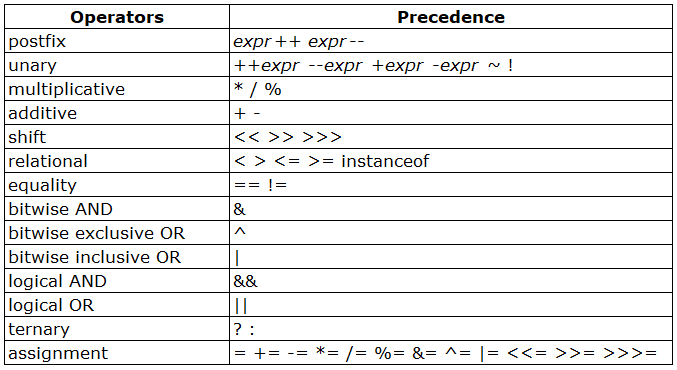# Operators In Java | Java Tutorial

In the previous post you have learned variables and data types. Now lets learn what we can do with them.

Operators in Java are the special symbols that perform specific operations and then return a result.

Types of Operators in Java are

1. Arithmetic Operators
2. Assignment Operators
3. Auto-increment Operator and Auto-decrement Operators
4. Logical Operators
5. Comparison (relational) Operators
6. Bitwise Operators
7. Ternary Operator

Arithmetic Operators:

Arithmetic operators are +, -, *, /, %

– is Subtraction
* is Multiplication
/ is Division
% is Modulus operator – it returns the remainder of an integer divisionAssignment Operators:

Assignments operators in java are: =, +=, -=, *=, /=, %=

Auto-increment and Auto-decrement Operators:

Auto-increment in Java is ++
Auto-decrement in Java is –

Logical Operators:

Logical operators in Java are &&, ||, !

Assume we have two boolean variables booleanVal1, booleanVal2

booleanVal1&&booleanVal2:

If booleanVal1 and booleanVal2 are true then output will be true else output will be false

booleanVal1||booleanVal2:

If booleanVal1 and booleanVal2 are false then output will be false else output will be true. Which means one of the value is true then it returns true.

!booleanVal1:

If the value of booleanVal1 is true then it returns false.

Comparison (relational) Operators:

Relational operators in Java are ==, !=, >, <, >=, <=

==      equal to – it returns true if both the left side and right side are equal
!=      not equal to – it returns true if left side is not equal to the right side of operator
>       greater than – it returns true if left side is greater than right
>=      greater than or equal to – it returns true if left side is greater than or equal to right side
<       less than – it returns true if left side is less than right side
<=      less than or equal to – it returns true if left side is less than or equal to right side

Bitwise Operators:

Bitwise Operators in Java are &, |, ^, ~, <<, >>

The bitwise & operator performs a bitwise AND operation.

The bitwise ^ operator performs a bitwise exclusive OR operation.

The bitwise | operator performs a bitwise inclusive OR operation.

The unary bitwise complement operator “~” inverts a bit pattern; it can be applied to any of the integral types, making every “0” a “1” and every “1” a “0”. For example, a byte contains 8 bits; applying this operator to a value whose bit pattern is “00000000” would change its pattern to “11111111”.

The signed left shift operator “<<” shifts a bit pattern to the left, and the signed right shift operator “>>” shifts a bit pattern to the right. The bit pattern is given by the left-hand operand, and the number of positions to shift by the right-hand operand. The unsigned right shift operator “>>>” shifts a zero into the leftmost position, while the leftmost position after “>>” depends on sign extension.

Ternary Operators:

Ternary operator evaluates a boolean expression and assign the value based on the result.

Syntax:

Variable num1 = (expression) ? value if expression is true : value if expression is false

Get our latest blog posts delivered to your inbox

Subscribe and get popular blog posts about software testing industry.YOU MAY ALSO ENJOY THESE ARTICLES